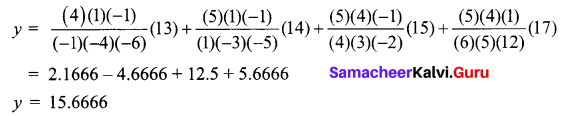# Samacheer Kalvi 12th Business Maths Solutions Chapter 5 Numerical Methods Additional Problems

One Mark Questions

Question 1.
Match the following.

 (a) ∆f(x) (i) 2x + 1 (b) E2 f(x) (ii) 1 + ∆ (c) E (iii) f(x + h) – f(x) (d) ∆x2, h = 1 (iv) f(x + 2h)

(a) – (iii), (b) – (iv), (c) – (ii), (d) – (i)

Question 2.
E-n f(x) is ______
(a) f(x + nh)
(b) f(x – nh)
(c) f(-nh)
(d) f(x – n)
(b) f(x – nh)Question 3.
E is a _____
(a) shifting operator
(b) Displacement operator
(c) 1 + ∆
(d) all of these
(e) none of these
(d) all of these

Question 4.
4 y3 = _______
(a) (E – 1)4 y3
(b) (E3 – 1) y3
(c) (E – 1)3 y0
(d) (E – 1)4 y0
(a) (E – 1)4 y3Question 5.
Fill in the blanks.

1. The two methods of interpolation are _______ and _______
2. If values of x are not equidistant we use _______ method.
3. ∆(f(x) + g(x)) = ______
4. k yn = ______
5. The first three terms in Newton’s method will give a ________ interpolation.

1. graphical method, algebraic method
2. Lagrange’s method
3. ∆f(x) + ∆g(x)
4. k-1 yn+1 – ∆k-1 yn
5. Parabolic

Question 6.
Say true or false

1. ∇y2 = y1 – y0
2. 2 yn = ∇yn – ∇yn+1
3. When 5 values are given, the polynomial which fits the data is of degree 4
4. E ∆ = ∆ E
5. f(2) + ∆f(2) = f(3)

1. False
2. True
3. True
4. True
5. True

II. 2 Mark Questions

Question 1.
Find the missing term from the following data.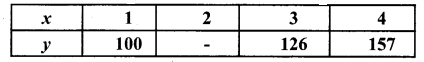Solution:
Since three values of y = f(x) are given, the polynomial which fits the data is of degree two.
Hence third differences are zero.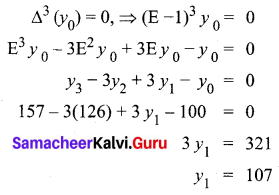Question 2.
From the following data estimate the export for the year 2000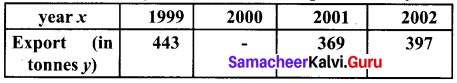Solution:
Consider a polynomial of degree two.
Hence third differences are zero.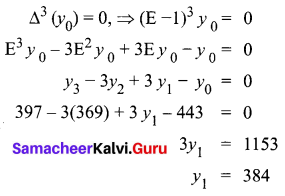Question 3.
For the tabulated values of y = f(x), find ∆y3 and ∆3y2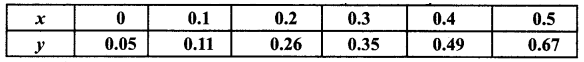Solution: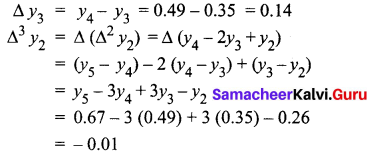Question 4.
If f(x) = x2 + ax + b, find ∆r f(x)
Solution:
∆f(x) = f(x + h) – f(x)
= [(x + h )2 + a(x + h) + b] – [x2 + ax + b]
= 2xh + h2 + ah
2 f(x) = [2(x + h) h + h2 + ah] – [2xh + h2 + ah] = 2h2
3 f(x) = 0
Thus ∆r f(x) = 0 for all r ≥ 3Question 5.
Show that ∆3 y4 = ∇3 y7
Solution: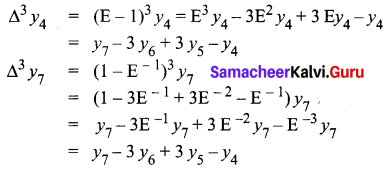Hence proved

III. 3 and 5 Marks Questions

Question 1.
If f(0) = 5, f(1) = 6, f(3) = 50, f(4) = 105, find f(2) by using Lagrange’s formula.
Solution: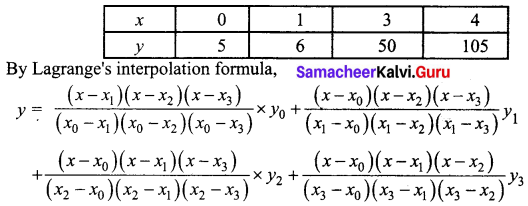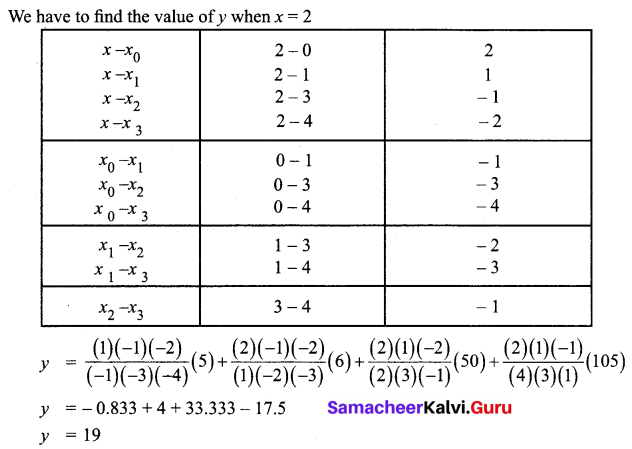Question 2.
Find y when x = 0.2 given that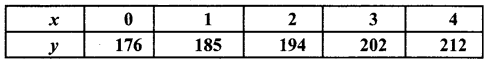Solution:
Since the required value of y is near the beginning of the table, we use Newton’s forward difference formula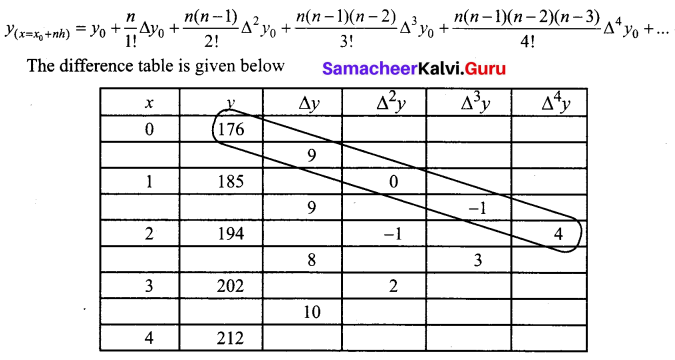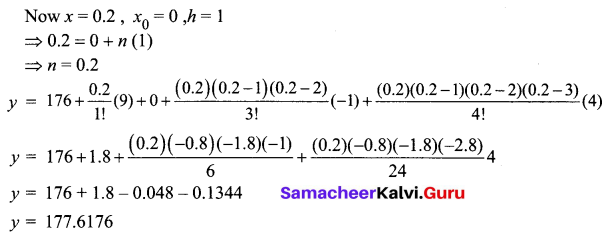Question 3.
Find the number of men getting wages between Rs.30 and Rs.35 from the following table: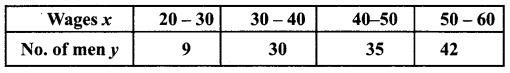Solution:
The difference table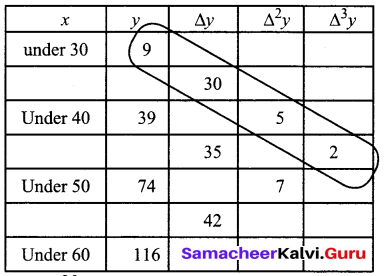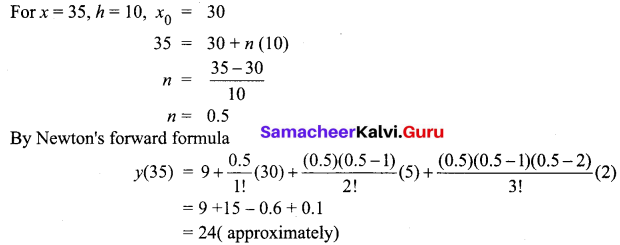No. of men getting wages less than 35 is 24. Therefore the number of men getting wages between Rs.30 and Rs.35 is y (35) – y (30)
(i.e) 24 – 9 = 15

Question 4.
Using Newton’s formula estimate the population of town for the year 1995: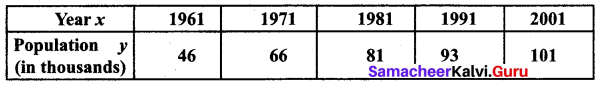Solution:
1995 lies in (1991, 2001). Hence we use Newton’s backward interpolation formula.
Here x = 1995, xn = 2001, h = 10
1995 = xn + nh
⇒ 1995 = 2001 + 10n
⇒ n = $$\frac{1995-2001}{10}$$
⇒ n = -0.6
The backward difference table is given below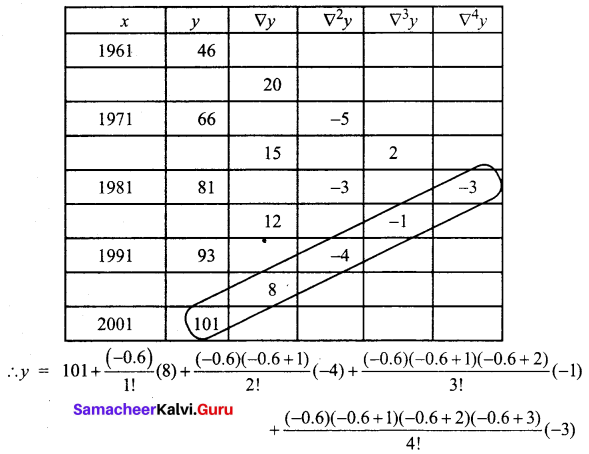y = 101 – 4.8 + 0.48 + 0.056 + 0.1008
y = 96.8368
Hence the population for the year 1995 is 96.837 thousands.Question 5.
Using Lagrange’s formula find y(11) from the following table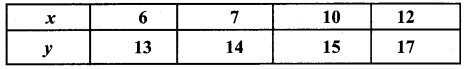Solution:
Given
x0 = 6, y0 = 13
x1 = 7, y1 = 14
x2 = 10, y2 = 15
x3 = 12, y3 = 17
x = 11
Using Lagrange’s formula,